## 背景和动机

2016年大概十月中下旬，同事推荐了一个视频：

https://netdevconf.org/1.2/session.html?yuchung-cheng

## 插曲

TCP BBR Startup gain计算总结和Startup失速问题https://blog.csdn.net/dog250/article/details/80780346

## 正文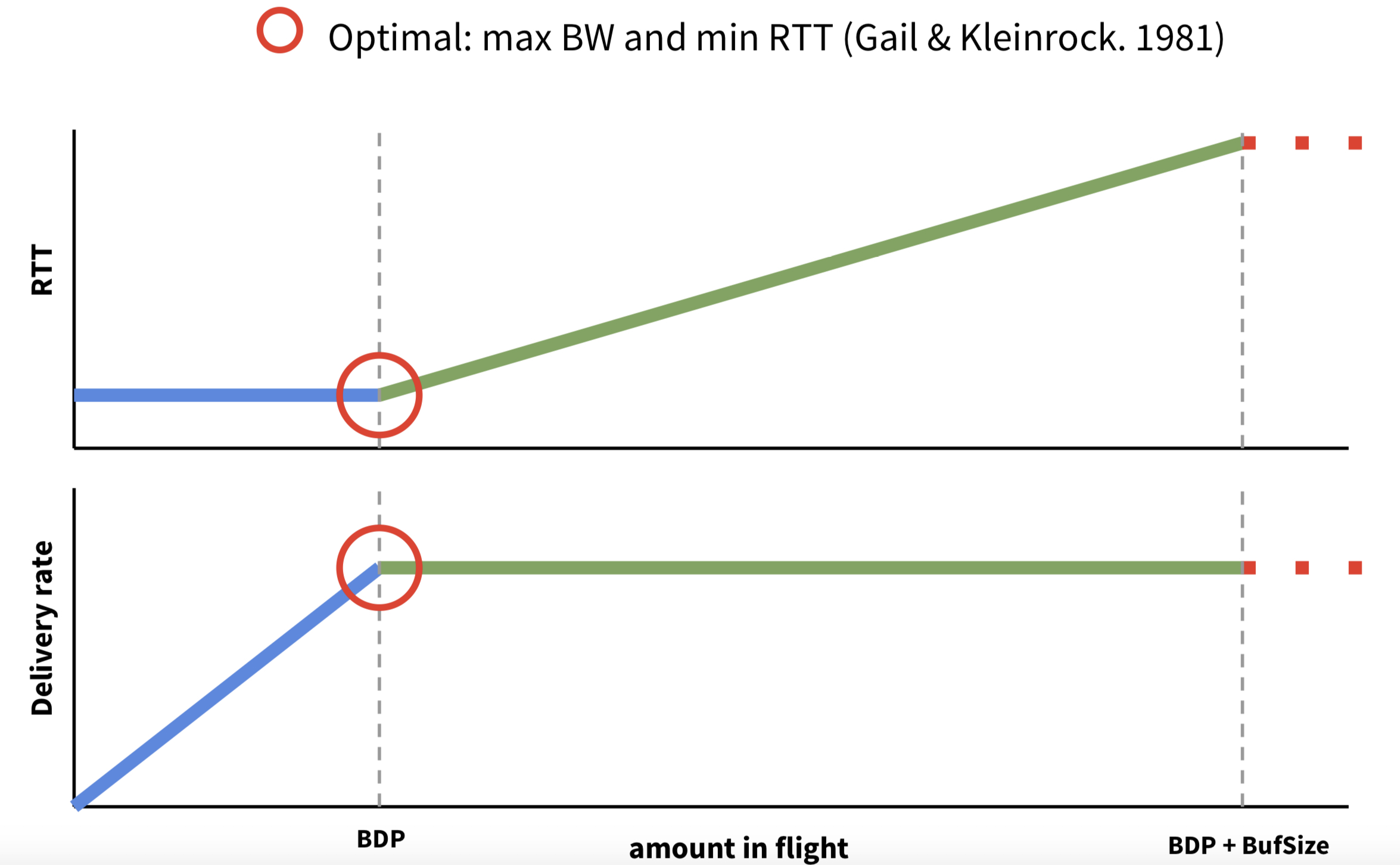BW： w w
RTT： t t
Inflight： f f

r = { 1 f ≤ f c f − f c − 1 f &gt; f c r = \begin{cases} 1 &amp; f \le f_c \\ f-f_c-1 &amp; f&gt;f_c \end{cases}

w = { f f c f ≤ f c 1 f &gt; f c w= \begin{cases} \dfrac{f}{f_c} &amp; f \le f_c \\ 1 &amp; f&gt;f_c \end{cases}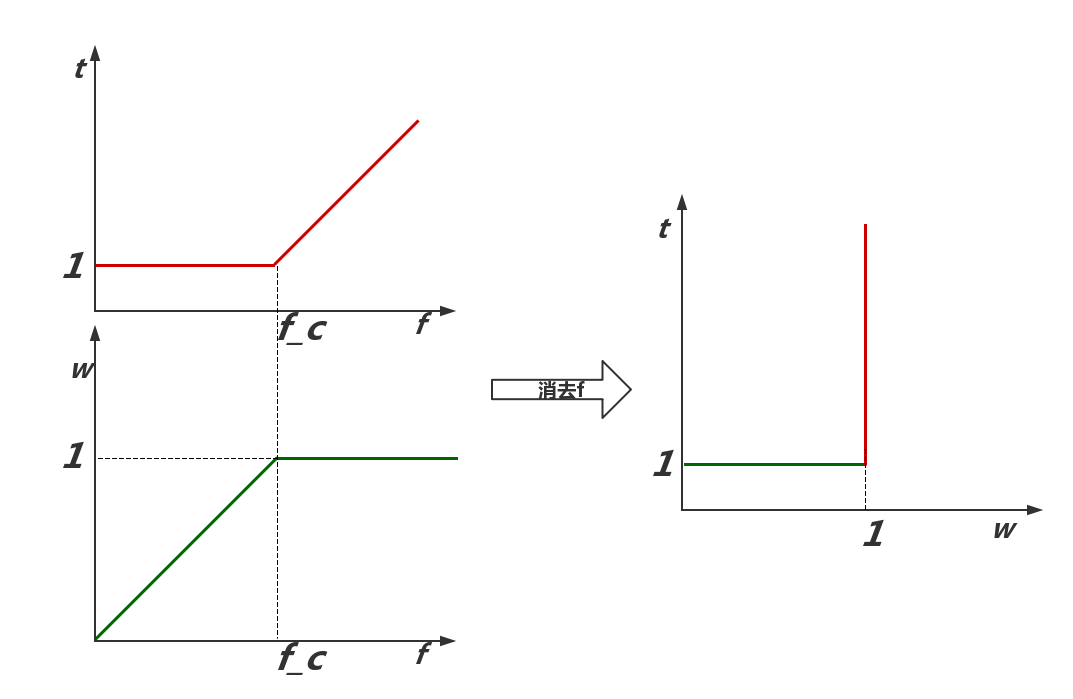E = w t E=\dfrac{w}{t}

f c f_c 时，系统的效能真的是最优的。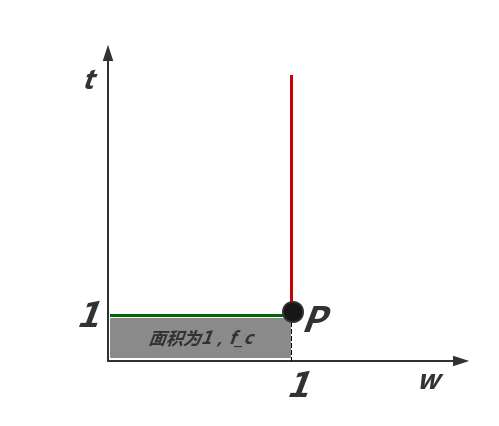1. 要么带宽没有用满，没有达到 w m a x w_{max} ;
2. 要么带宽超额了，数据排队了，数据到达的太快，因此延迟增加了；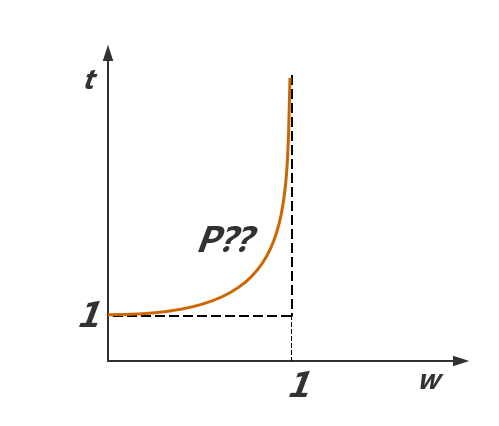t = f ( w ) t=f(w)

E = w t = w f ( w ) E=\dfrac{w}{t}=\dfrac{w}{f(w)}

E ′ = w × d f ( w ) d w − f ( w ) f 2 ( w ) E\prime=\dfrac{w\times \dfrac{d f(w)}{d w}-f(w)}{f^2(w)}

E ′ = w × d f ( w ) d w − f ( w ) f 2 ( w ) = 0 E\prime=\dfrac{w\times \dfrac{d f(w)}{d w}-f(w)}{f^2(w)}=0
f ( w ) = w × d f ( w ) d w f(w)=w\times \dfrac{d f(w)}{d w}

f ( w ) w = d f ( w ) d w \dfrac{f(w)}{w}=\dfrac{d f(w)}{dw}

• 左边： f ( w ) f(w) 上任意一点到原点的直线的斜率；
• 右边：曲线 f ( w ) f(w) 上任意一点切线的斜率。

• 到达率： λ \lambda
• 服务率： μ \mu
• 系统负荷水平： ρ \rho
• 用户停留时间： W s W_s

• 系统负荷水平
ρ = λ μ \rho=\dfrac{\lambda}{\mu}
• 用户停留时间(包括排队时间和被服务的时间)
W s = 1 μ − λ W_s=\dfrac{1}{\mu-\lambda}
• 当前系统中用户数(包括排队的和正在被服务的)
L s = λ μ − λ L_s=\dfrac{\lambda}{\mu-\lambda}
• 排队等待时间
W g = λ μ × ( μ − λ ) W_g=\dfrac{\lambda}{\mu \times (\mu-\lambda)}

R T T = f ( λ ) = W s = 1 μ − λ = 1 C − λ RTT=f(\lambda)=W_s=\dfrac{1}{\mu-\lambda}=\dfrac{1}{C-\lambda}

f ( λ ) = λ × d f ( λ ) d λ f(\lambda)=\lambda \times \dfrac{d f(\lambda)}{d \lambda}

f ( λ ) = λ × d f ( λ ) d λ = 1 C − λ f(\lambda)=\lambda\times \dfrac{d f(\lambda)}{d \lambda}=\dfrac{1}{C-\lambda}

d f ( λ ) d λ = 1 ( C − λ ) 2 \dfrac{d f(\lambda)}{d\lambda}=\dfrac{1}{(C-\lambda)^2}

λ ( C − λ ) 2 = 1 C − λ \dfrac{\lambda}{(C-\lambda)^2}=\dfrac{1}{C-\lambda}
λ = C − λ \lambda=C-\lambda
λ = C 2 \lambda=\dfrac{C}{2}

P ( C 2 , 2 C ) P(\dfrac{C}{2},\dfrac{2}{C})

L s = λ μ − λ = C 2 C − C 2 = 1 L_s=\dfrac{\lambda}{\mu-\lambda}=\dfrac{\dfrac{C}{2}}{C-\dfrac{C}{2}}=1

B D P = λ × f ( λ ) = C 2 × 2 C = 1 BDP=\lambda\times f(\lambda)=\dfrac{C}{2}\times \dfrac{2}{C}=1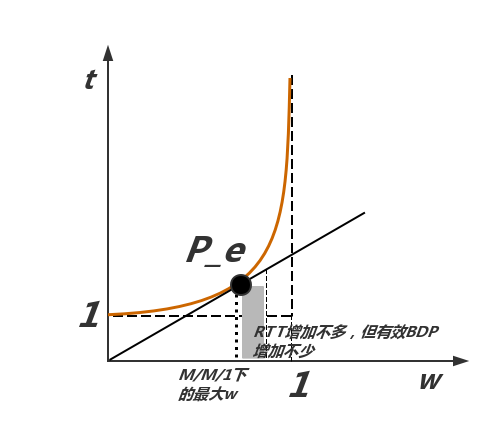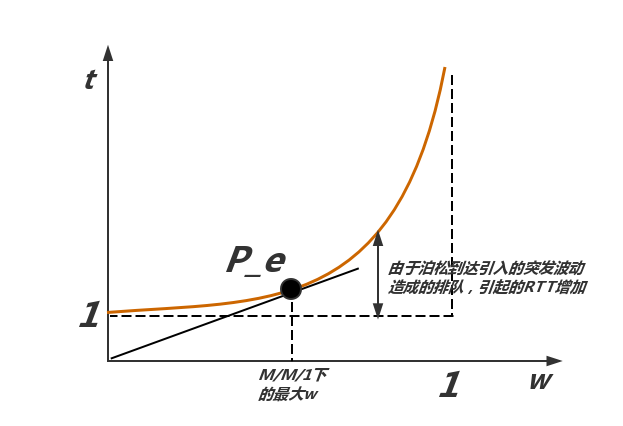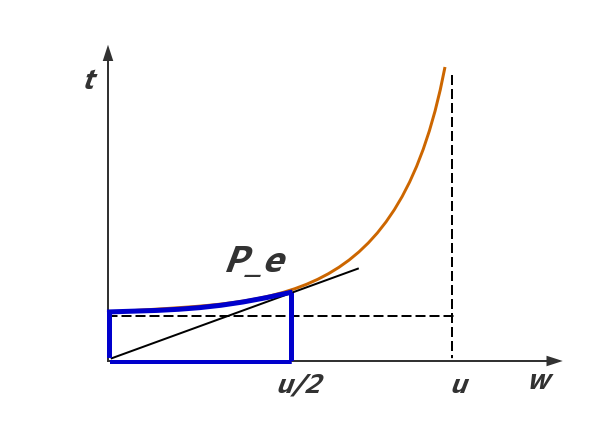## 宏观背景下的BBR

1980年代的拥塞崩溃导致了1980年代的拥塞控制机制的出炉，某种意义上这属于见招拆招的策略，针对1980年代的拥塞，提出了1980年代的拥塞控制算法，即ss，ssthresh，congestion avoid这些。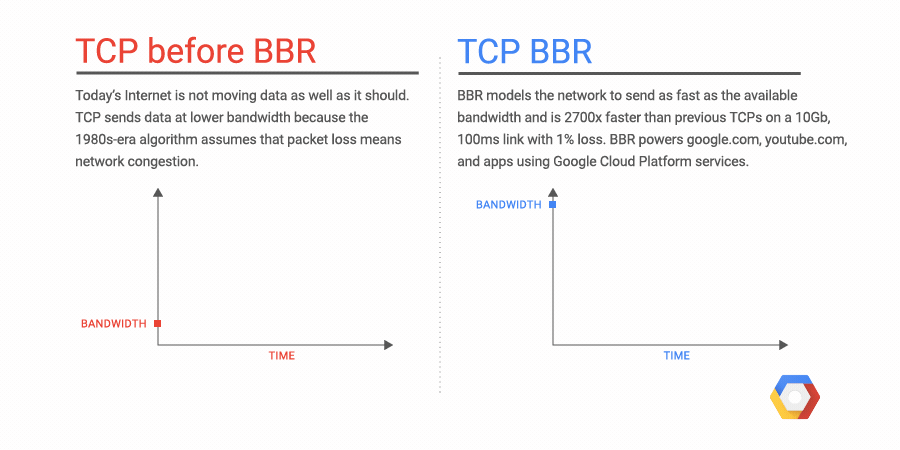## 附录：关于排队论一些典型结论的推导

https://zh.wikipedia.org/zh-hans/等候理論

• 没有用户排队
• 有1个用户排队
• 有2个用户排队
• 有3个用户排队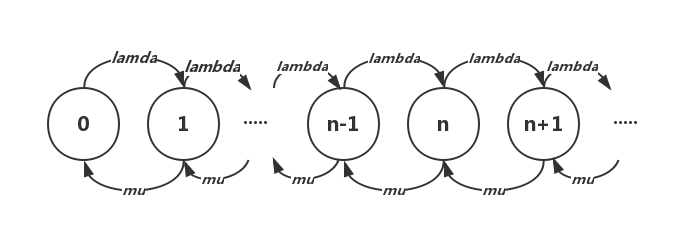λ P n − 1 + μ P n + 1 = λ P n + μ P n \lambda P_{n-1}+\mu P_{n+1}=\lambda P_n+\mu P_n
λ P 0 = μ P 1 \lambda P_0 = \mu P_1

{ λ P 0 = μ P 1 λ P n − 1 + μ P n + 1 = λ P n + μ P n n ≥ 1 \begin{cases} \lambda P_0 = \mu P_1 \\ \lambda P_{n-1}+\mu P_{n+1}=\lambda P_n+\mu P_n &amp; n \ge 1 \end{cases}

1. 朝着左边雪崩：用户和任务将会被清空，资源浪费；
2. 朝着右边雪崩：系统完全过载而崩溃，系统不再可用。

{ P 0 = 1 − λ μ P n = ( λ μ ) n ( 1 − λ μ ) n ≥ 1 \begin{cases} P_0 = 1-\dfrac{\lambda}{\mu} \\ P_n=(\dfrac{\lambda}{\mu})^n(1-\dfrac{\lambda}{\mu}) &amp; n \ge 1 \end{cases}

{ P 0 = 1 − ρ P n = ρ n P 0 n ≥ 1 \begin{cases} P_0 = 1-\rho \\ P_n=\rho^nP_0 &amp; n \ge 1 \end{cases}

N = Σ n = 0 ∞ n P n = . . . 动 用 微 积 分 吧 . . . = ρ 1 − ρ = λ μ − λ N=\Sigma_{n=0}^\infty nP_n=...动用微积分吧...=\dfrac{\rho}{1-\rho}=\dfrac{\lambda}{\mu-\lambda}

04-042万+
04-30
09-221389
10-222万+
02-161523
01-04951
08-078319
11-23726
05-291689
10-212万+
10-10975
12-29420
10-231万+

### “相关推荐”对你有帮助么？

•非常没帮助
•没帮助
•一般
•有帮助
•非常有帮助被折叠的  条评论 为什么被折叠?到【灌水乐园】发言dog250

¥2 ¥4 ¥6 ¥10 ¥20余额支付 (余额：-- )扫码支付获取中扫码支付点击重新获取扫码支付1.余额是钱包充值的虚拟货币，按照1:1的比例进行支付金额的抵扣。
2.余额无法直接购买下载，可以购买VIP、C币套餐、付费专栏及课程。余额充值